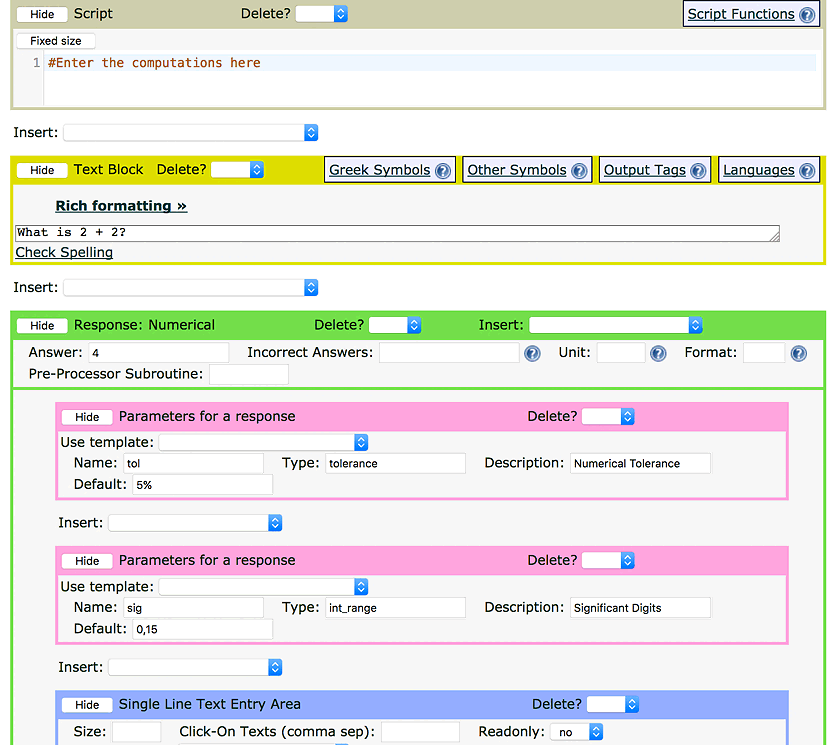###LON-CAPA HelpFigure 1: Numerical Response editor

A Numerical Response problem has seven major parts by default:

1. The Script is the heart of advanced Numerical Response problems. It can be used to decide some of the parameters of the problem, compute the answer to the problem, and do just about anything else you can imagine. The Script language is Perl. You do not need to know Perl to use the Script block because we will be stepping through some advanced examples in this chapter, but knowing Perl can help.

2. Like other problem types, the Text Block is used to display the problem the student will see. In addition, you can place variables in the Text Block based on computations done in the Script.

3. The Answer is the answer the system is looking for. The answer can use variables calculated/defined in the problem's Script block, allowing the answer to be determined dynamically (including randomization).